Updating search results...

# 29 Results

View
Selected filters:
• CCSS.Math.Content.6.EE.A.1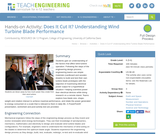Educational Use
Rating
0.0 stars

Students gain an understanding of the factors that affect wind turbine operation. Following the steps of the engineering design process, engineering teams use simple materials (cardboard and wooden dowels) to build and test their own turbine blade prototypes with the objective of maximizing electrical power output for a hypothetical situation—helping scientists power their electrical devices while doing research on a remote island. Teams explore how blade size, shape, weight and rotation interact to achieve maximal performance, and relate the power generated to energy consumed on a scale that is relevant to them in daily life. A PowerPoint® presentation, worksheet and post-activity test are provided.

Subject:
Career and Technical Education
Mathematics
Measurement and Data
Numbers and Operations
Physical Science
Material Type:
Activity/Lab
Provider:
TeachEngineering
Author:
Alexander Kon
02/07/2017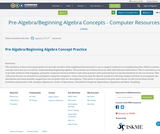Conditional Remix & Share Permitted
CC BY-NC-SA
Rating
4.0 stars

The intention of this curriculum guide is to provide teachers with supplemental materials to use to support students in strengthening their skills in various concept areas that are crucial for understanding beginning algebra. The activities are broken down by skill with links provided below. This is intended as a way to provide students with engaging, primarily computer-based activities to get extra practice with material that is covered elsewhere in the curriculum. This collection focuses on simulations and games using the computer—some resources may be ripe for teachers to develop unique activities to accompany the simulation and some possible suggestions are included with the descriptions. This series is intended to be pick-and-choose.

In this Curriculum Guide:

Activities and practice with: Integers, Exponents, Order of Operations, Distributive Property, Expressions, Equations and Basic Graphing

Subject:
Mathematics
Material Type:
Activity/Lab
Diagram/Illustration
Game
Interactive
Simulation
Author:
Lee Gargagliano
05/24/2018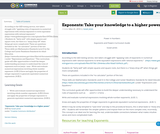Unrestricted Use
CC BY
Rating
4.0 stars

According to the GED testing service, test takers struggle with “applying rules of exponents in numerical expressions with rational exponents to write equivalent expressions with rational exponents.” (https://www.gedtestingservice.com/uploads/files/09738c12fe4e4accd9a16bab7cb99a3c.pdf )
Students do “fairly well” with simple squares and square roots, but there is a “sharp drop-off” when things get more complicated.
These are questions included in the “no calculator” portion of the test.
These skills are Mathematics Standards Level D in the College and Career Readiness Standards for Adult Education (https://www.educateiowa.gov/sites/files/ed/documents/CCRStandardsAdultEd.pdf ) under “Expressions and Equations.”
This curriculum guide will offer opportunities to build the deeper understanding necessary to understand the rules of exponents such as (xm)n = xmn .
Write and evaluate numerical expressions involving whole-number exponents. (6.EE.1)
Know and apply the properties of integer exponents to generate equivalent numerical expressions. (8.EE.1)

Subject:
Mathematics
Material Type:
Activity/Lab
Lesson Plan
Teaching/Learning Strategy
Author:
Susan Jones
05/25/2018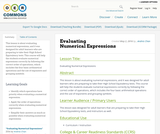Unrestricted Use
CC BY
Rating
0.0 stars

This lesson is about evaluating numerical expressions, and it was designed for adult learners who are preparing to take their High School Equivalency tests. This course will help the students evaluate numerical expressions correctly by following the correct order of operations, which includes the four basic arithmetical operations and the use of exponents and grouping symbols.

Subject:
Mathematics
Material Type:
Lesson
Author:
Andres Chan
01/17/2017Conditional Remix & Share Permitted
CC BY-NC
Rating
5.0 stars

This unit is an EQuIP Exemplar for adult education (http://achieve.org/equip). Students will connect their prior, real-world knowledge to the concept of order in mathematics. They will go through a discovery process with content that will build a deep, conceptual understanding of the properties of operations to explain why we perform operations in a certain order when we see just the naked numbers.

Material Type:
Lesson Plan
Unit of Study
Provider:
EQuIP Exemplars
Author:
Connie Rivera
11/26/2014Unrestricted Use
CC BY
Rating
0.0 stars

The purpose of this task is to introduce the idea of exponential growth and then connect that growth to expressions involving exponents. It illustrates well how fast exponential expressions grow.

Subject:
Mathematics
Material Type:
Activity/Lab
Provider:
Illustrative Mathematics
Provider Set:
Illustrative Mathematics
Author:
Illustrative Mathematics
05/01/2012Only Sharing Permitted
CC BY-NC-ND
Rating
4.0 stars

This lesson unit is intended to help you assess how well students are able to: Perform arithmetic operations, including those involving whole-number exponents, recognizing and applying the conventional order of operations; Write and evaluate numerical expressions from diagrammatic representations and be able to identify equivalent expressions; apply the distributive and commutative properties appropriately; and use the method for finding areas of compound rectangles.

Subject:
Geometry
Material Type:
Assessment
Lesson Plan
Provider:
Shell Center for Mathematical Education
Provider Set:
Mathematics Assessment Project (MAP)
04/26/2013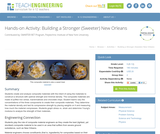Educational Use
Rating
0.0 stars

Students create and analyze composite materials with the intent of using the materials to construct a structure with optimal strength and minimal density. The composite materials are made of puffed rice cereal, marshmallows and chocolate chips. Student teams vary the concentrations of the three components to create their composite materials. They determine the material density and test its compressive strength by placing weights on it and measuring how much the material compresses. Students graph stress vs. strain and determine Young's modulus to analyze the strength of their materials.

Subject:
Architecture and Design
Engineering
Material Type:
Activity/Lab
Provider:
TeachEngineering
Provider Set:
TeachEngineering
Author:
Charisse Nelson
Sarah Wigodsky
10/14/2015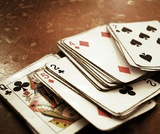Conditional Remix & Share Permitted
CC BY-NC
Rating
4.0 stars

Students explore what makes a math trick work by analyzing verbal math expressions that describe each step in the trick.Key ConceptsWords can be used to describe mathematical operations.In a math trick, a person starts with a number, follows mathematical directions given in words, and ends up with the original number.Math tricks can be explained by examining the mathematical expressions that represent the verbal directions.Goals and Learning ObjectivesExplore verbal expressions.Predict and test which sets of expressions will result in the original number.

Subject:
Mathematics
Material Type:
Lesson Plan
Provider:
Pearson
09/21/2015Conditional Remix & Share Permitted
CC BY-NC
Rating
0.0 stars

Subject:
Mathematics
Material Type:
Full Course
Provider:
Pearson
10/06/2016Rating
0.0 stars

Getting Started

Type of Unit: Introduction

Prior Knowledge

Students should be able to:

Solve and write numerical equations for whole number addition, subtraction, multiplication, and division problems.
Use parentheses to evaluate numerical expressions.
Identify and use the properties of operations.

Lesson Flow

In this unit, students are introduced to the rituals and routines that build a successful classroom math community and they are introduced to the basic features of the digital course that they will use throughout the year.

An introductory card sort activity matches students with their partner for the week. Then over the course of the week, students learn about the lesson routines: Opening, Work Time, Ways of Thinking, Apply the Learning, Summary of the Math, and Reflection. Students learn how to present their work to the class, the importance of taking responsibility for their own learning, and how to effectively participate in the classroom math community.

Students then work on Gallery problems to further explore the program’s technology resources and tools and learn how to organize their work.

The mathematical work of the unit focuses on numerical expressions, including card sort activities in which students identify equivalent expressions and match an expression card to a word card that describes its meaning. Students use the properties of operations to identify equivalent expressions and to find unknown values in equations.

Subject:
Mathematics
Material Type:
Unit of Study
Provider:
PearsonRating
0.0 stars

Expressions

Type of Unit: Concept

Prior Knowledge

Students should be able to:

Write and evaluate simple expressions that record calculations with numbers.
Use parentheses, brackets, or braces in numerical expressions and evaluate expressions with these symbols.
Interpret numerical expressions without evaluating them.

Lesson Flow

Students learn to write and evaluate numerical expressions involving the four basic arithmetic operations and whole-number exponents. In specific contexts, they create and interpret numerical expressions and evaluate them. Then students move on to algebraic expressions, in which letters stand for numbers. In specific contexts, students simplify algebraic expressions and evaluate them for given values of the variables. Students learn about and use the vocabulary of algebraic expressions. Then they identify equivalent expressions and apply properties of operations, such as the distributive property, to generate equivalent expressions. Finally, students use geometric models to explore greatest common factors and least common multiples.

Subject:
Mathematics
Algebra
Material Type:
Unit of Study
Provider:
Pearson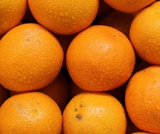Conditional Remix & Share Permitted
CC BY-NC
Rating
0.0 stars

Students are introduced to classroom routines and expectations, and complete a full mathematics lesson. The class discusses how to clearly present work to classmates. Partner work is modeled, and partners then work to match numerical expressions to corresponding word descriptions. Students read and discuss a summary of the math in the lesson, and then write a reflection about their thoughts.Key ConceptsStudents match a numerical expression to its corresponding description in words. Students interpret parentheses and brackets in numerical expressions and they construct viable arguments and critique the reasoning of others. Students learn to use the exponent 2 to represent squaring.Goals and Learning ObjectivesDescribe the classroom routines and expectations.Consider how to present work clearly to classmates.Collaborate with a partner.Critique a partner’s reasoning.Connect a numerical expression to its corresponding word description.Learn to use an exponent of 2 to represent squaring.

Subject:
Algebra
Material Type:
Lesson Plan
Provider:
Pearson
09/21/2015Unrestricted Use
CC BY
Rating
0.0 stars

This is a task from the Illustrative Mathematics website that is one part of a complete illustration of the standard to which it is aligned. Each task has at least one solution and some commentary that addresses important asects of the task and its potential use. Here are the first few lines of the commentary for this task: Take a square with area 1. Divide it into 9 equal-sized squares. Remove the middle one. What is the area of the figure now? Take the remaining 8 square...

Subject:
Mathematics
Material Type:
Activity/Lab
Provider:
Illustrative Mathematics
Provider Set:
Illustrative Mathematics
Author:
Illustrative Mathematics
08/06/2013Conditional Remix & Share Permitted
CC BY-NC
Rating
0.0 stars

Students analyze how two different calculators get different values for the same numerical expression. In the process, students recognize the need for following the same conventions when evaluating expressions.Key ConceptsMathematical expressions express calculations with numbers (numerical expressions) or sometimes with letters representing numbers (algebraic expressions).When evaluating expressions that have more than one operation, there are conventions—called the order of operations—that must be followed:Complete all operations inside parentheses first.Evaluate exponents.Then complete all multiplication and division, working from left to right.Then complete all addition and subtraction, working from left to right.These conventions allow expressions with more than one operation to be evaluated in the same way by everyone. Because of these conventions, it is important to use parentheses when writing expressions to indicate which operation to do first. If there are nested parentheses, the operations in the innermost parentheses are evaluated first. Understanding the use of parentheses is especially important when interpreting the associative and the distributive properties.Goals and Learning ObjectivesEvaluate numerical expressions.Use parentheses when writing expressions.Use the order of operations conventions.

Subject:
Algebra
Material Type:
Lesson Plan
Provider:
Pearson
09/21/2015Conditional Remix & Share Permitted
CC BY-NC
Rating
0.0 stars

Students review the ways classroom habits and routines can strengthen their mathematical character. Students learn what a Gallery is and how to choose a Gallery problem to work on. They then choose one of three Gallery problems that introduce the unit’s technology resources. The three Gallery problems combine working with expressions with the resources available with this unit.Key ConceptsUnderstand that a Gallery gives students a choice of several problems. Understand what to consider when choosing a problem. Know how to work on a Gallery problem and how to present work on gallery problems.Goals and Learning ObjectivesKnow how to choose a problem from a Gallery.

Subject:
Mathematics
Material Type:
Lesson Plan
Provider:
Pearson
09/21/2015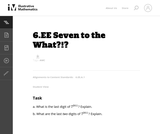Unrestricted Use
CC BY
Rating
0.0 stars

This is a task from the Illustrative Mathematics website that is one part of a complete illustration of the standard to which it is aligned. Each task has at least one solution and some commentary that addresses important asects of the task and its potential use. Here are the first few lines of the commentary for this task: What is the last digit of \$7^{2011}\$? Explain. What are the last two digits of \$7^{2011}\$? Explain....

Subject:
Mathematics
Material Type:
Activity/Lab
Provider:
Illustrative Mathematics
Provider Set:
Illustrative Mathematics
Author:
Illustrative Mathematics
05/19/2013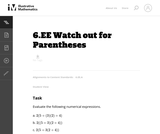Unrestricted Use
CC BY
Rating
0.0 stars

This problem asks the student to evaluate three numerical expressions that contain the same integers yet have differing results due to placement of parentheses.

Subject:
Mathematics
Material Type:
Activity/Lab
Provider:
Illustrative Mathematics
Provider Set:
Illustrative Mathematics
Author:
Illustrative Mathematics
08/27/2012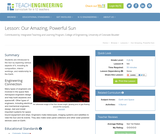Educational Use
Rating
0.0 stars

The purpose of this lesson is to introduce the students to the Sun. They explore various aspects of the Sun including its composition, its interior workings, and its relationship to the Earth.

Subject:
Engineering
Astronomy
Material Type:
Activity/Lab
Lesson Plan
Provider:
TeachEngineering
Provider Set:
TeachEngineering
Author:
Brian Kay
Geoffrey Hill
Janet Yowell
Jessica Butterfield
Jessica Todd